### Home > PC > Chapter 1 > Lesson 1.1.4 > Problem1-51

1-51.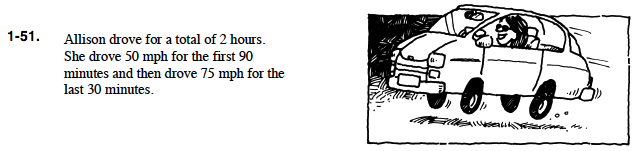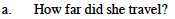What is distance in terms of rate and time? Be careful with the units. Convert minutes to hours since the rate is in miles per hour.
The first 90 minutes is started below. Finish the calculations.
50(1.5) + ...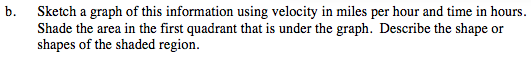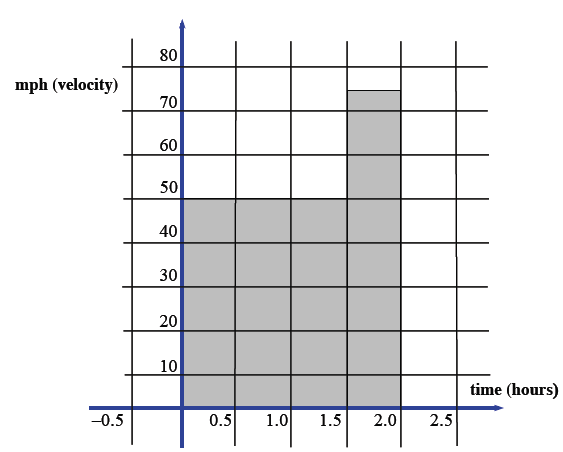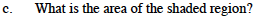The shaded region is two triangles. What is the area of each triangle?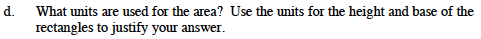What are the units of the x-axis and the y-axis?

$\frac{\text{miles}}{\text{hour}} \cdot \text{hours}$